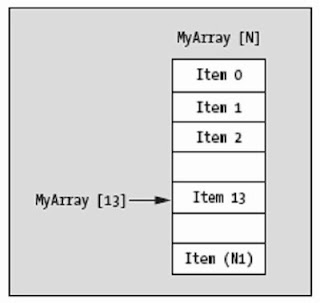# Arrays in Java: how to declare, use and access its elements

In this introductory article of Arrays of our online Progressive Java course, you will learn how to declare and use arrays, or vectors, in Java.

Learn, too, the important notation of brackets, [], which we use to treat the members of an Array, as well as access the elements through these brackets.

## Declaring Arrays (vectors) in Java

The syntax of the declaration and creation of the array is as follows:
type[]  arrayName = new type[number_of_elements];

Or:
type[]  arrayName = { value1, value2, ..., valueN};

In the last case, the size of the array is the size of elements between the braces {}.

For example, some classroom has 20 students and you want to declare 20 variables of type float using array would look like this:

float[] score = new int ;
float[] score = {1,2,3,4,5,6,7,8,90.1,10.02, -11,12.9,13.7,14.5,15.7,16.0,17.5,19.3,20.2};

And the name of the students, armazenaríamos in Strings:
String[] name = new String ;

If you notice well, we're using the word 'new', which is the same we use in Scanner class, to create an object. Here is not different. We are creating an object of arrays as well.

Two important information:

1. When you declare variables using numeric array and not initialized with values, these will be automatically void, 0 or 0.0.
If they are Strings, will be 'null' and empy if they are char.

2. The array size is constant. Once declared, its value can not be changed.
If declared 5 integers, the array will always have 5 size .

If you want to know the size of an array, use the 'length', which is in every object of type array and returns an integer with the number of elements in each array each: name.length, scor.length etc.

## The order of the elements into the Array

Let us now show important information, not only from Java, but programming.In our day-to-day we count: 1, 2, 3 ...
Do not count using the 0.

In programming, obviously that includes Java, we ALWAYS start counting at 0!

For example, if you declare an array named 'test' of 3 elements, its elements will be identified by:
test 
test 
test 

Therefore, whenever we declare an array of 'n' indices, the index these values ​​range from 0 to n-1.
Save it, is important and always will use from now on.

## Using the elements of an array in Java

Okay, you declared an array / vector. It has elements of type float. But used only one name, 'score'.
How to represent 20 variables with only one name?
The answer is simple: using numbers or index.

'score' is an Array of floats.
If you want to use a float type, use the following syntax: name[index]

So your variables, independently, are called: score. score, score etc..
These are their names. You can use as you would variables, for example:

Storing a note from a student who take 10
score = 10.0 // this is probably Java programmer

Adding a note of two students:
float sum = score + score;

Increase:
score++;

Anyway, you can do everything. Are variables of type float normal.
The difference is that the variable names have numbers that are called, in programming, the index, which are created automatically when you declare a block of several elements.

### Examples of Java code using Array

Example 1: Write a program that asks the user to three integers, store in an Array, then show the value of each element of the array, as well as its content.

Step 1: First we declare an array of integers containing 3 elements:
int[] score = new int ;

After, create the object 'input' of type scanner, for receiving the user values.

Step 2: Store the values ​​in array
Note that you are programmer and knows that the indices range from 0 to 2.
But you do not. To user, is number 1, number 2 and number 3, does not start at 0.

In the loop, our 'index' goes from 0 to 2.
However, to receive the value of index 'index', we are asking the user the number 'index+1' .

For example, to store a value in 'note ', we will ask for the number '0 +1' to user.
To store a value in 'note', we will ask for the number '1 +1' to user.
To store a value in 'note', we will ask for the number '2 +1' to user.

Step 3: Displaying the values to user
We will use another loop to display the value of the indices, ranging from 0 to 2.
But again, we have to show 1-3 to user because it does not make sense to 'number 0 -> value 10',  but '1 -> value 10'.

So our Java code illustrating the use of arrays is:

``````import java.util.Scanner;

public class arrayTest {

public static void main(String[] args){
int[] score = new int;
Scanner input = new Scanner(System.in);

//receiving the numbers
for(int index=0 ; index < 3 ; index++){
System.out.print("\nType the number " + (index+1) + ": ");
score[index] = input.nextInt();
}

//displaying the numbers
for(int index=0 ; index < 3 ; index++){
System.out.printf("Number %d -> Stored value: %d\n",index+1, score[index]);
}
}

}
``````

Example 2: Write a Java application that asks the student's name, get two scores and then returns all that information along with the average scores.

Let's use this example, an array of floats with 3 elements.
Two elements for storing the values ​​of the note and the third will store the average.

The rest, no secret. Here's how our Java code:

``````import java.util.Scanner;

public class arrayTest2 {

public static void main(String[] args){
float[] score = new float;
String name;
Scanner input = new Scanner(System.in);

System.out.print("Student name: ");
name = input.nextLine();

System.out.print("Score 1: ");
score = input.nextFloat();

System.out.print("Score 2: ");
score = input.nextFloat();

//average
score = (score + score)/2;

System.out.printf("The student '%s' got %.1f and %.1f, so the average is %.2f",name,score,score,score);

}

}
``````

#### 1 comment:Anonymous said...

float[] score = new int ;
did you mean:
float[] score = new float ;